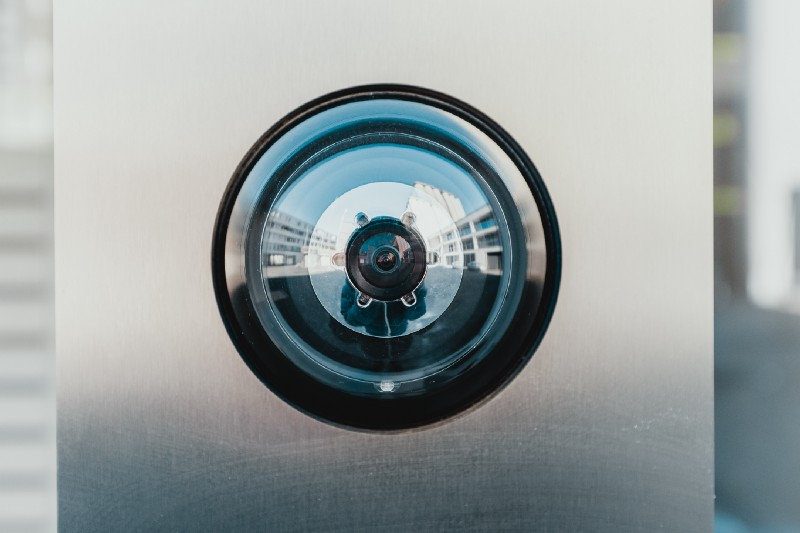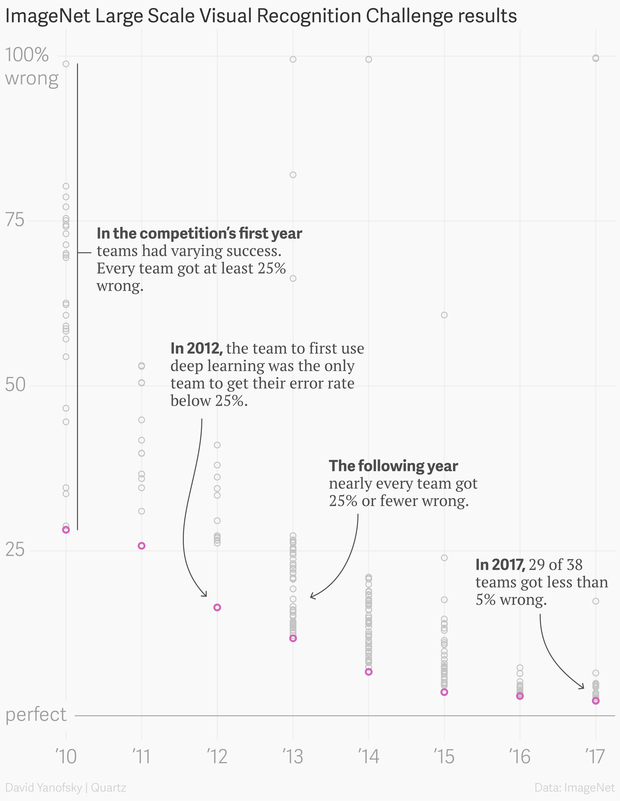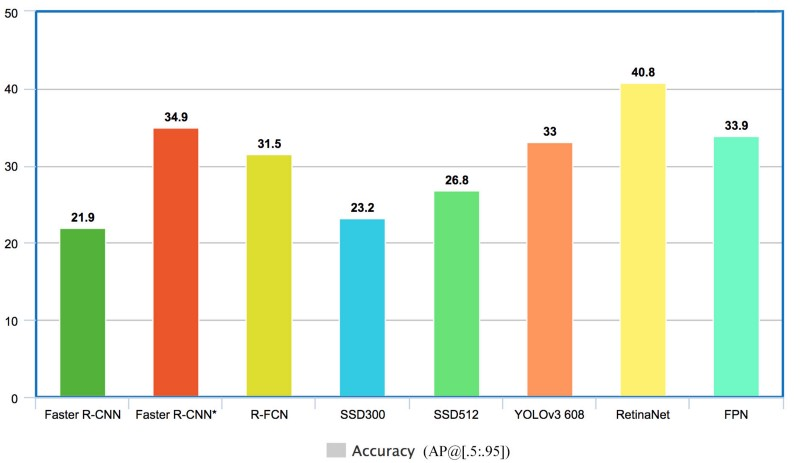# Simple Surveillance System with the Tensorflow Object Detection APISurveillance is an integral part of security and is used all over the world to ensure the safety of valuables as well as people.

Even though surveillance is controversial in some situations. Especially when connected to surveillance of human beings it is necessary for other tasks like watching over valuables, monitoring operations, ensuring employee safety as well as loss prevention and public safety.

Surveillance, mostly, is a repetitive mostly trivial tasks which raises the question:

Can machines possibly automate surveillance?

This is a question a lot of people not familiar with deep learning might have on their mind.

Anyone with a little knowledge of deep learning and computer vision can probably tell them that deep learning has surpassed human performance on most computer vision task.Figure 2: Error on Image Classification with the ImageNet data-set(Source)

The same progress was also achieved in the domain of object detection so yes, a deep learning models is more that capable to detect the right objects in images most of the time.Figure 3: Object Detection model scores on the COCO data-set (source)

### How can we implement a simple surveillance system ourselves?

So now that we know that surveillance, for the most part, can be automated using machine learning we might wonder how we can build our own simple surveillance system.

Building a ‘small’ surveillance system is quite simple. We only need to train a object detection model on the classes we want to detect and then react when an object is detected.

In this article, we will simply save an image of the detected object and record the time it was detected into a csv file.

For creating the object detection model we will be using the Tensorflow Object Detection API.

The Tensorflow Object Detection API is an open source framework that allows you to use pretrained object detection models or create and train new models by making use of transfer learning. This is extremely useful because building an object detection model from scratch can be difficult and can take a very long time to train.

If you haven’t installed the Tensorflow Object Detection API yet you can check out my article covering how to do so.

### Recap on how to use the Tensorflow Object Detection API for Live Object Detection

In my article called “Live Object Detection” I covered how to rewrite the Tensorflow Object Detection demo script to work with a live video stream. For this we rewrote the run_inference_for_single_image method to only include the code that needs to be executed once and moved all the other code outside the method.

def run_inference_for_single_image(image, graph):    if 'detection_masks' in tensor_dict:        # The following processing is only for single image        detection_boxes = tf.squeeze(tensor_dict['detection_boxes'], )        detection_masks = tf.squeeze(tensor_dict['detection_masks'], )        # Reframe is required to translate mask from box coordinates to image coordinates and fit the image size.        real_num_detection = tf.cast(tensor_dict['num_detections'], tf.int32)        detection_boxes = tf.slice(detection_boxes, [0, 0], [real_num_detection, -1])        detection_masks = tf.slice(detection_masks, [0, 0, 0], [real_num_detection, -1, -1])        detection_masks_reframed = utils_ops.reframe_box_masks_to_image_masks(            detection_masks, detection_boxes, image.shape, image.shape)        detection_masks_reframed = tf.cast(            tf.greater(detection_masks_reframed, 0.5), tf.uint8)        # Follow the convention by adding back the batch dimension        tensor_dict['detection_masks'] = tf.expand_dims(            detection_masks_reframed, 0)    image_tensor = tf.get_default_graph().get_tensor_by_name('image_tensor:0')    # Run inference    output_dict = sess.run(tensor_dict,                            feed_dict={image_tensor: np.expand_dims(image, 0)})    # all outputs are float32 numpy arrays, so convert types as appropriate    output_dict['num_detections'] = int(output_dict['num_detections'])    output_dict['detection_classes'] = output_dict[        'detection_classes'].astype(np.uint8)    output_dict['detection_boxes'] = output_dict['detection_boxes']    output_dict['detection_scores'] = output_dict['detection_scores']    if 'detection_masks' in output_dict:        output_dict['detection_masks'] = output_dict['detection_masks']    return output_dict  import cv2cap = cv2.VideoCapture(0)try:    with detection_graph.as_default():        with tf.Session() as sess:                # Get handles to input and output tensors                ops = tf.get_default_graph().get_operations()                all_tensor_names = {output.name for op in ops for output in op.outputs}                tensor_dict = {}                for key in [                  'num_detections', 'detection_boxes', 'detection_scores',                  'detection_classes', 'detection_masks'                ]:                    tensor_name = key + ':0'                    if tensor_name in all_tensor_names:                        tensor_dict[key] = tf.get_default_graph().get_tensor_by_name(                      tensor_name)                while True:                    ret, image_np = cap.read()                    # Expand dimensions since the model expects images to have shape: [1, None, None, 3]                    image_np_expanded = np.expand_dims(image_np, axis=0)                    # Actual detection.                    output_dict = run_inference_for_single_image(image_np, detection_graph)                    # Visualization of the results of a detection.                    vis_util.visualize_boxes_and_labels_on_image_array(                        image_np,                        output_dict['detection_boxes'],                        output_dict['detection_classes'],                        output_dict['detection_scores'],                        category_index,                        instance_masks=output_dict.get('detection_masks'),                        use_normalized_coordinates=True,                        line_thickness=8)                    cv2.imshow('object_detection', cv2.resize(image_np, (800, 600)))                    if cv2.waitKey(25) & 0xFF == ord('q'):                        cap.release()                        cv2.destroyAllWindows()                        breakexcept Exception as e:    print(e)    cap.release()

This code is a useful starting point but we aren’t yet accessing the individual objects. Furthermore because I will just use a model trained on the coco data-set I will also create a variable that specifies what objects we are looking for so we can check if an object has that class.

### Get the coordinates and labels for each box

We can access the label and coordinates of the objects using the detection_classes and detection_boxes keys for the output_dict dictionary.

output = []for index, score in enumerate(output_dict['detection_scores']):    if score < threshold:        continue    label = category_index[output_dict['detection_classes'][index]]['name']    ymin, xmin, ymax, xmax = output_dict['detection_boxes'][index]    output.append((label, int(xmin * image_width), int(ymin * image_height), int(xmax * image_width), int(ymax * image_height)))

In the code above we loop through all the scores for the detected objects. If the score of the object is higher than the specified threshold we will get the label and coordinates and append them to our output array. If the score is lower than the threshold we will just skip the object.

### Check if the box contains a human

To now react on some classes we can simply loop through the output array and check if the label corresponds to the class we are looking for.

If the label matches the class we will cut out the part of the image that contains the class and save it as a jpg. We will also save the datetime and filepath into a csv so we know the time when the image was taken.

for l, x_min, y_min, x_max, y_max in output:    if l == label_to_look_for:        array = cv2.cvtColor(np.array(image_show), cv2.COLOR_RGB2BGR)        image = Image.fromarray(array)        cropped_img = image.crop((x_min, y_min, x_max, y_max))        file_path = output_directory+'/images/'+str(len(df))+'.jpg'        cropped_img.save(file_path, "JPEG", icc_profile=cropped_img.info.get('icc_profile'))        df.loc[len(df)] = [datetime.datetime.now(), file_path]        df.to_csv(output_directory+'/results.csv', index=None)

### Putting things together

Now the only thing left to do is to but all the pieces together. We will also create a method for loading in the data-set and use the argparse library so we can pass the path of the model, labelmap, threshold, output directory and the class we want to search for.

import argparsefrom PIL import Imageimport cv2import numpy as npimport osimport pandas as pdimport datetimeimport tensorflow as tfimport syssys.path.append("C:/Users/Gilbert/Desktop/Programming/TensorflowModels/research/slim")sys.path.append("C:/Users/Gilbert/Desktop/Programming/TensorflowModels/research/object_detection")from utils import visualization_utils as vis_utilfrom object_detection.utils import ops as utils_opsfrom utils import label_map_utildef run_inference_for_single_image(image, sess, tensor_dict):    if 'detection_masks' in tensor_dict:        # The following processing is only for single image        detection_boxes = tf.squeeze(tensor_dict['detection_boxes'], )        detection_masks = tf.squeeze(tensor_dict['detection_masks'], )        # Reframe is required to translate mask from box coordinates to image coordinates and fit the image size.        real_num_detection = tf.cast(tensor_dict['num_detections'], tf.int32)        detection_boxes = tf.slice(detection_boxes, [0, 0], [real_num_detection, -1])        detection_masks = tf.slice(detection_masks, [0, 0, 0], [real_num_detection, -1, -1])        detection_masks_reframed = utils_ops.reframe_box_masks_to_image_masks(            detection_masks, detection_boxes, image.shape, image.shape)        detection_masks_reframed = tf.cast(            tf.greater(detection_masks_reframed, 0.5), tf.uint8)        # Follow the convention by adding back the batch dimension        tensor_dict['detection_masks'] = tf.expand_dims(detection_masks_reframed, 0)    image_tensor = tf.get_default_graph().get_tensor_by_name('image_tensor:0')    # Run inference    output_dict = sess.run(tensor_dict, feed_dict={image_tensor: np.expand_dims(image, 0)})    # all outputs are float32 numpy arrays, so convert types as appropriate    output_dict['num_detections'] = int(output_dict['num_detections'])    output_dict['detection_classes'] = output_dict[        'detection_classes'].astype(np.uint8)    output_dict['detection_boxes'] = output_dict['detection_boxes']    output_dict['detection_scores'] = output_dict['detection_scores']    if 'detection_masks' in output_dict:        output_dict['detection_masks'] = output_dict['detection_masks']    return output_dictdef load_model(PATH_TO_FROZEN_GRAPH: str, PATH_TO_LABELS: str):    """    Load model from frozen inference graph    """    # Load frozen inference graph into memory    graph = tf.Graph()    with graph.as_default():        od_graph_def = tf.GraphDef()        with tf.gfile.GFile(PATH_TO_FROZEN_GRAPH, 'rb') as fid:            serialized_graph = fid.read()            od_graph_def.ParseFromString(serialized_graph)            tf.import_graph_def(od_graph_def, name='')    # Loading labelmap    category_index = label_map_util.create_category_index_from_labelmap(PATH_TO_LABELS, use_display_name=True)    return graph, category_indexdef run_surveillance(model_path, labelmap_path, show_video_steam, label_to_look_for, output_directory, threshold):    # Load model    graph, category_index = load_model(model_path, labelmap_path)    # Initialize Video Capture    cap = cv2.VideoCapture(0)    # Create output directory if not already created    os.makedirs(output_directory, exist_ok=True)    os.makedirs(output_directory+'/images', exist_ok=True)    if os.path.exists(output_directory+'/results.csv'):        df = pd.read_csv(output_directory+'/results.csv')    else:        df = pd.DataFrame(columns=['timestamp', 'img_path'])    # Open detection graph    with graph.as_default():        with tf.Session() as sess:            # Get handles to input and output tensors            ops = tf.get_default_graph().get_operations()            all_tensor_names = {output.name for op in ops for output in op.outputs}            tensor_dict = {}            for key in [                'num_detections', 'detection_boxes', 'detection_scores',                'detection_classes', 'detection_masks'            ]:                tensor_name = key + ':0'                if tensor_name in all_tensor_names:                    tensor_dict[key] = tf.get_default_graph().get_tensor_by_name(tensor_name)            while True:                try:                    # Read frame from video                    ret, image_np = cap.read()                    # Copy image for later                    image_show = np.copy(image_np)                    image_height, image_width, _ = image_np.shape                    # Actual detection.                    output_dict = run_inference_for_single_image(image_np, sess, tensor_dict)                    if show_video_steam:                        # Visualize results                        vis_util.visualize_boxes_and_labels_on_image_array(                            image_np,                            output_dict['detection_boxes'],                            output_dict['detection_classes'],                            output_dict['detection_scores'],                            category_index,                            instance_masks=output_dict.get('detection_masks'),                            use_normalized_coordinates=True,                            line_thickness=8)                        cv2.imshow('object_detection', cv2.resize(image_np, (800, 600)))                        if cv2.waitKey(25) & 0xFF == ord('q'):                            cap.release()                            cv2.destroyAllWindows()                            break                    # Get data(label, xmin, ymin, xmax, ymax)                    output = []                    for index, score in enumerate(output_dict['detection_scores']):                            if score < threshold:                                continue                            label = category_index[output_dict['detection_classes'][index]]['name']                            ymin, xmin, ymax, xmax = output_dict['detection_boxes'][index]                            output.append((label, int(xmin * image_width), int(ymin * image_height), int(xmax * image_width), int(ymax * image_height)))                    # Save incident (could be extended to send a email or something)                    for l, x_min, y_min, x_max, y_max in output:                        if l == label_to_look_for:                            array = cv2.cvtColor(np.array(image_show), cv2.COLOR_RGB2BGR)                            image = Image.fromarray(array)                            cropped_img = image.crop((x_min, y_min, x_max, y_max))                            file_path = output_directory+'/images/'+str(len(df))+'.jpg'                            cropped_img.save(file_path, "JPEG", icc_profile=cropped_img.info.get('icc_profile'))                            df.loc[len(df)] = [datetime.datetime.now(), file_path]                            df.to_csv(output_directory+'/results.csv', index=None)                except Exception as e:                    print(e)                    cap.release()                    cv2.destroyAllWindows()                    breakif __name__ == '__main__':    parser = argparse.ArgumentParser(description='Surveillance System')    parser.add_argument('-m', '--model_path', type=str, required=True, help='Path to the frozen inference graph')    parser.add_argument('-l', '--labelmap', type=str, required=True, help='Path to labelmap')    parser.add_argument('-t', '--threshold', type=float, default=0.5, help='Threshold for bounding boxes')    parser.add_argument('-s', '--show', default=True, action='store_true', help='Show window')    parser.add_argument('-la', '--label', default='person', type=str, help='Label name to detect')    parser.add_argument('-o', '--output_directory', default='results', type=str, help='Directory for the outputs')    args = parser.parse_args()    run_surveillance(args.model_path, args.labelmap, args.show, args.label, args.output_directory, args.threshold)

We can test this script by typing

python simple_surveillance_system.py -m <path_to_model> -l <path_to_labelmap>

You can also specify any of the other parameters but you don’t need to because all of them have preset values.

### Use ImUtils to allow for support of the Raspberry Camera

Another thing we can to is to add support for the Raspberry Camera. We can to so using the imutils library.

Imutils is build on top of OpenCV and offers a lot of convenience functions to make basic image processing easier. It includes functions for translating, rotating, resizing images as well as many more.

To incorporate ImUtils in our script we only need to change the initialization of the camera, the reading of a frame as well as the closing of the camera when exiting the script.

import argparsefrom PIL import Imagefrom imutils.video import VideoStreamimport cv2import numpy as npimport osimport pandas as pdimport datetimeimport tensorflow as tfimport syssys.path.append("C:/Users/Gilbert/Desktop/Programming/TensorflowModels/research/slim")sys.path.append("C:/Users/Gilbert/Desktop/Programming/TensorflowModels/research/object_detection")from utils import visualization_utils as vis_utilfrom object_detection.utils import ops as utils_opsfrom utils import label_map_utildef run_inference_for_single_image(image, sess, tensor_dict):    if 'detection_masks' in tensor_dict:        # The following processing is only for single image        detection_boxes = tf.squeeze(tensor_dict['detection_boxes'], )        detection_masks = tf.squeeze(tensor_dict['detection_masks'], )        # Reframe is required to translate mask from box coordinates to image coordinates and fit the image size.        real_num_detection = tf.cast(tensor_dict['num_detections'], tf.int32)        detection_boxes = tf.slice(detection_boxes, [0, 0], [real_num_detection, -1])        detection_masks = tf.slice(detection_masks, [0, 0, 0], [real_num_detection, -1, -1])        detection_masks_reframed = utils_ops.reframe_box_masks_to_image_masks(            detection_masks, detection_boxes, image.shape, image.shape)        detection_masks_reframed = tf.cast(            tf.greater(detection_masks_reframed, 0.5), tf.uint8)        # Follow the convention by adding back the batch dimension        tensor_dict['detection_masks'] = tf.expand_dims(detection_masks_reframed, 0)    image_tensor = tf.get_default_graph().get_tensor_by_name('image_tensor:0')    # Run inference    output_dict = sess.run(tensor_dict, feed_dict={image_tensor: np.expand_dims(image, 0)})    # all outputs are float32 numpy arrays, so convert types as appropriate    output_dict['num_detections'] = int(output_dict['num_detections'])    output_dict['detection_classes'] = output_dict[        'detection_classes'].astype(np.uint8)    output_dict['detection_boxes'] = output_dict['detection_boxes']    output_dict['detection_scores'] = output_dict['detection_scores']    if 'detection_masks' in output_dict:        output_dict['detection_masks'] = output_dict['detection_masks']    return output_dictdef load_model(PATH_TO_FROZEN_GRAPH: str, PATH_TO_LABELS: str):    """    Load model from frozen inference graph    """    # Load frozen inference graph into memory    graph = tf.Graph()    with graph.as_default():        od_graph_def = tf.GraphDef()        with tf.gfile.GFile(PATH_TO_FROZEN_GRAPH, 'rb') as fid:            serialized_graph = fid.read()            od_graph_def.ParseFromString(serialized_graph)            tf.import_graph_def(od_graph_def, name='')    # Loading labelmap    category_index = label_map_util.create_category_index_from_labelmap(PATH_TO_LABELS, use_display_name=True)    return graph, category_indexdef run_surveillance(model_path, labelmap_path, show_video_steam, label_to_look_for, output_directory, threshold, picamera):    # Load model    graph, category_index = load_model(model_path, labelmap_path)    # Initialize Video Stream    vs = VideoStream(usePiCamera=picamera, resolution=(640, 480)).start()    # Create output directory if not already created    os.makedirs(output_directory, exist_ok=True)    os.makedirs(output_directory+'/images', exist_ok=True)    if os.path.exists(output_directory+'/results.csv'):        df = pd.read_csv(output_directory+'/results.csv')    else:        df = pd.DataFrame(columns=['timestamp', 'img_path'])    # Open detection graph    with graph.as_default():        with tf.Session() as sess:            # Get handles to input and output tensors            ops = tf.get_default_graph().get_operations()            all_tensor_names = {output.name for op in ops for output in op.outputs}            tensor_dict = {}            for key in [                'num_detections', 'detection_boxes', 'detection_scores',                'detection_classes', 'detection_masks'            ]:                tensor_name = key + ':0'                if tensor_name in all_tensor_names:                    tensor_dict[key] = tf.get_default_graph().get_tensor_by_name(tensor_name)            while True:                try:                    # Read frame from video                    image_np = vs.read()                    # Copy image for later                    image_show = np.copy(image_np)                    image_height, image_width, _ = image_np.shape                    # Actual detection.                    output_dict = run_inference_for_single_image(image_np, sess, tensor_dict)                    if show_video_steam:                        # Visualize results                        vis_util.visualize_boxes_and_labels_on_image_array(                            image_np,                            output_dict['detection_boxes'],                            output_dict['detection_classes'],                            output_dict['detection_scores'],                            category_index,                            instance_masks=output_dict.get('detection_masks'),                            use_normalized_coordinates=True,                            line_thickness=8)                        cv2.imshow('object_detection', cv2.resize(image_np, (800, 600)))                        if cv2.waitKey(25) & 0xFF == ord('q'):                            vs.stop()                            cv2.destroyAllWindows()                            break                    # Get data(label, xmin, ymin, xmax, ymax)                    output = []                    for index, score in enumerate(output_dict['detection_scores']):                            if score < threshold:                                continue                            label = category_index[output_dict['detection_classes'][index]]['name']                            ymin, xmin, ymax, xmax = output_dict['detection_boxes'][index]                            output.append((label, int(xmin * image_width), int(ymin * image_height), int(xmax * image_width), int(ymax * image_height)))                    # Save incident (could be extended to send a email or something)                    for l, x_min, y_min, x_max, y_max in output:                        if l == label_to_look_for:                            array = cv2.cvtColor(np.array(image_show), cv2.COLOR_RGB2BGR)                            image = Image.fromarray(array)                            cropped_img = image.crop((x_min, y_min, x_max, y_max))                            file_path = output_directory+'/images/'+str(len(df))+'.jpg'                            cropped_img.save(file_path, "JPEG", icc_profile=cropped_img.info.get('icc_profile'))                            df.loc[len(df)] = [datetime.datetime.now(), file_path]                            df.to_csv(output_directory+'/results.csv', index=None)                except Exception as e:                    print(e)                    vs.stop()                    cv2.destroyAllWindows()                    breakif __name__ == '__main__':    parser = argparse.ArgumentParser(description='Surveillance System')    parser.add_argument('-m', '--model_path', type=str, required=True, help='Path to the frozen inference graph')    parser.add_argument('-l', '--labelmap', type=str, required=True, help='Path to labelmap')    parser.add_argument('-t', '--threshold', type=float, default=0.5, help='Threshold for bounding boxes')    parser.add_argument('-s', '--show', default=True, action='store_true', help='Show window')    parser.add_argument('-la', '--label', default='person', type=str, help='Label name to detect')    parser.add_argument('-o', '--output_directory', default='results', type=str, help='Directory for the outputs')    parser.add_argument('--picamera', action='store_true', help='Use PiCamera for image capture', default=False)    args = parser.parse_args()    run_surveillance(args.model_path, args.labelmap, args.show, args.label, args.output_directory, args.threshold, args.picamera)

### Can we trust our model

Being able to trust a model is really important especially when the model is used to make important decisions like whether to let someone lend money.

In order to be sure that our model only detects humans when their really is a human in the picture we need to test. We can do so by simply running our model and watching if it does the right thing but we can also use model interpretation tools to help us understand our model.

If you want to learn more about model interpretation and why it is important you can check out my article series on model interpretation.

### Summary

Today machine learning models are capable of automating repetitive trivial tasks like image classification or object detection. The only thing they need to do so is lots of data.

A Surveillance System can be build using a object detection model that monitors the desired classes.

### What’s next?

In the upcoming months I will blog about different applications of machine learning and data science as well as some new technologies and libraries. Furthermore, I will focus more on learning the theory behind machine learning in depth.

That’s all from this article. If you have any questions or just want to chat with me feel free to leave a comment below or contact me on social media. If you want to get continous updates about my blog make sure to follow me on Medium and join my newsletter.